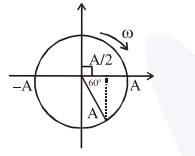# Y = A sin (ωt + Φ0) is the time-displacement equation`
Question:

$\mathrm{Y}=\mathrm{A} \sin \left(\omega \mathrm{t}+\phi_{0}\right)$ is the time-displacement equation of a SHM. At $t=0$ the displacement

of the particle is $Y=\frac{A}{2}$ and it is moving along

negative $x$-direction. Then the initial phase angle $\phi_{0}$ will be :

1. $\frac{\pi}{6}$

2. $\frac{\pi}{3}$

3. $\frac{5 \pi}{6}$

4. $\frac{2 \pi}{3}$

Correct Option: , 3

Solution:initial phase $\frac{\pi}{2}+\frac{\pi}{3}=\frac{5 \pi}{6}$